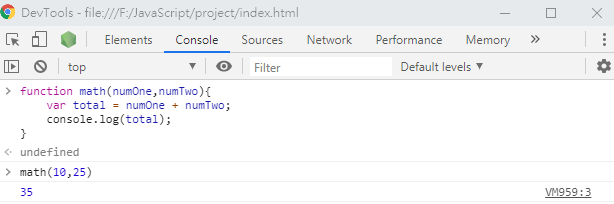#0

## 從 0 學習 JavaScript 筆記－在函式 function 加入參數# 函式帶入參數

``````function number() {
console.log('Tim') //字串要加引號
console.log(323) //數字不用加引號

}

number(); //執行函式
``````

### 練習帶入一個參數會這樣寫：

``````function math(number) {
var total = number; //宣告變數的值等於參數
console.log(total) //驗證結果是變數的名稱，但這邊不是輸入字串，故不用加上引號

}

math(10); //執行函式，並帶入參數
``````

### 函式 + 參數 + 運算

EX. 加法

``````function math(numOne, numTwo) {
var total = numOne + numTwo //結果為兩個變數相加
console.log(total) //驗證變數
}

math(10,25); //執行函式，並帶入參數
``````